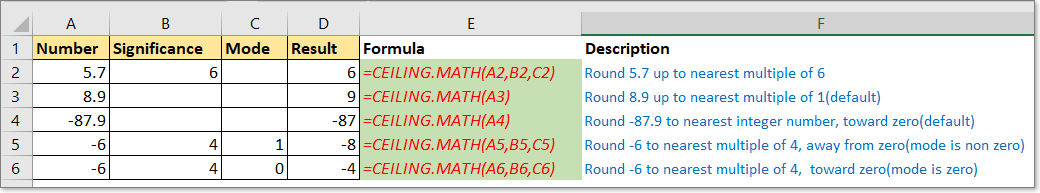## Excel CEILING.MATH function### Description

The CEILING.MATH function rounds a given number up to the nearest multiple of significance or to the nearest integer number.

### Syntax and arguments

Formula syntax

 =CEILING.MATH(number, [significance],[mode])

Arguments

 Number: Required. The number value you want to round up. Significance: Optional. The multiple you want to round number to. Mode: Optional. Decide to round negative numbers toward or away from zero while using the CEILING.MATH function, and default is 0.

Return Value

The CEILING.MATH function rounds number up to nearest multiple.

Notes:

1. If the significance is omitted or default, the significance is 1 for positive number, -1 for negative number. For example, 2 rounded to 2, -2 rounded to -2.

2. If the significance is omitted or default, the positive decimal numbers will be rounded up to nearest integer. For instance, round 1.23 to 2.

3. If the significance and mode are omitted or default, the negative decimal numbers will be rounded up to nearest integer which is toward to zero. For instance, round -1.23 to -1.

4. Both of the number and significance are negative, the result is rounded down away from zero.

5. If the Mode is non-zero, the negative number will be round up to nearest multiple of significance away from zero, for instance, =CEILING.MATH(-12,5,1), rounds -12 to -15.

6. If the number is an exact multiple of significance, no changes occurs.

### Usage and Examples

Here are some examples provided for you to understand how to use the CEILING.MATH function in Excel.

Positive integer number

 Number Significance Mode Result Formula Notes 8 8 =CEILING.MATH(8) Round 8 up to the nearest multiple of 1(default) 8 11 11 =CEILING.MATH(8,11) Round 8 up to the nearest multiple of 11

Positive decimal number

 Number Significance Mode Result Formula Notes 1.23 2 =CEILING.MATH(1.23) Round 1.23 up to the nearest multiple of 1(default) 1.23 3 3 =CEILING.MATH(1.23,3) Round 1.23 up to the nearest multiple of 3

Positive integer number

 Number Significance Mode Result Formula Notes -10 -10 =CEILING.MATH(-10) Round -10 up to the nearest multiple of 1(default), toward to zero(default) -10 3 -9 =CEILING.MATH(-10,3) Round -10 up to the nearest multiple of 3, toward to zero(default) -10 3 1 -12 =CEILING.MATH(-10,3,1) Round -10 to the nearest multiple of 3, away from zero

Negative decimal number

 Number Significance Mode Result Formula Notes -22.1 -21 =CEILING.MATH(-21.11) Round -21.11 up to the nearest multiple of 1(default), toward to zero(default) -22.1 10 -20 =CEILING.MATH(-21.11,10) Round -21.11 up to the nearest multiple of 10, toward to zero(default) -22.1 10 1 -30 =CEILING.MATH(-21.11,10,1) Round -21.11 to the nearest multiple of 10, away from zero

#### Relative Functions:

• Excel CEILING Function
The CEILING function returns a number rounded up to a nearest multiple of significance. For example, =CEILING(10.12,0.2) returns 10.2, it round 10.12 to nearest multiple of 0.2.

• Excel CEILING.PRECISE Function
The CEILING.PRECISE function returns a number rounded up to a nearest multiple or nearest integer ignoring sign of number, it round all numbers up.

### The Best Office Productivity Tools

#### Kutools for Excel - Helps You To Stand Out From Crowd

 Popular Features: Find/Highlight/Identify Duplicates  |  Delete Blank Rows  |  Combine Columns or Cells without Losing Data  |  Round without Formula ... Super VLookup: Multiple Criteria  |  Multiple Value   |  Across Multi-Sheets  |  Fuzzy Lookup... Adv. Drop-down List: Easy Drop Down List  |  Dependent Drop Down List  |  Multi-select Drop Down List... Column Manager: Add a Specific Number of Columns  |  Move Columns  |  Unhide Columns  |  Compare Columns to Select Same & Different Cells ... Featured Features: Grid Focus  |  Design View  |  Big Formula Bar  |  Workbook & Sheet Manager  |  Resource Library (Auto Text)  |  Date Picker  |  Combine Worksheets  |  Encrypt/Decrypt Cells  |  Send Emails by List  |  Super Filter  |  Special Filter (filter bold/italic/strikethrough...) ... Top 15 Toolset:  12 Text Tools (Add Text, Remove Characters ...)  |  50+ Chart Types (Gantt Chart ...)  |  40+ Practical Formulas (Calculate age based on birthday ...)  |  19 Insertion Tools (Insert QR Code, Insert Picture from Path ...)  |  12 Conversion Tools (Numbers to Words, Currency Conversion ...)  |  7 Merge & Split Tools (Advanced Combine Rows, Split Cells ...)  |  Many More...

Kutools for Excel Boasts Over 300 Features, Ensuring That What You Need is Just A Click Away...

Supports Office/Excel 2007-2021 and 365   |   Available in 44 Languages   |   Full-Featured 30-Day Free Trial.#### Office Tab - Enable Tabbed Reading and Editing in Microsoft Office (include Excel)

• One second to switch between dozens of open documents!
• Reduce hundreds of mouse clicks for you every day, say goodbye to mouse hand.
• Increases your productivity by 50% when viewing and editing multiple documents.
• Brings Efficient Tabs to Office (include Excel), Just Like Chrome, Firefox, And New Internet Explorer.